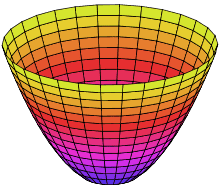I read that concave mirrors are part of a sphere, and concave mirrors can also be expressed in a parabola equation, but a parabola equation is expressed as #4py=x^2# and a circle as #x^2+y^2=R^2#. So the two can't be the same right? Can someone please explain this? Thank you in advance :)

phinds
Gold Member
2021 Award
I read that concave mirrors are part of a sphere, and concave mirrors can also be expressed in a parabola equation, but a parabola equation is expressed as #4py=x^2# and a circle as #x^2+y^2=R^2#. So the two can't be the same right?
Right. Perhaps you mis-read one of them or perhaps there are two different kinds of mirrors. If only one is right which one do you think it would be?

Actually, concave isn't related to a particular shape. Concave just means hollowed inwards.
Are both the cases you mentioned hollowed inwards?

phinds
Gold Member
2021 Award
Actually, concave isn't related to a particular shape. Concave just means hollowed inwards.
Good point

Drakkith
Staff Emeritus
The basic shape of a curved mirror is a conic section rotated around an axis to form a surface. (A conic section being a slice of a cone at various angles) A parabolic mirror is shaped like parabola that has been rotated. This surface is called a paraboloid, specifically an elliptic paraboloid.You can think of the mirror as a small portion of the very bottom of this shape.

Rotating other conic surfaces, such as ellipses, circles, and hyperbolas, yields a differently shaped surface for each. More complicated mirrors can have a very complex surface shape that isn't simply a rotated conic section. We even have adaptive optics in professional telescopes that actively change the shape of the mirror to compensate for the effect turbulence in the atmosphere has on incoming light.

http://en.wikipedia.org/wiki/Conic_section
http://en.wikipedia.org/wiki/Parabolic_reflector

•bubblewrap
phinds
Gold Member
2021 Award
Drakkith, that certainly true but it is just your opinion that a mirror is automatically a parabolic surface? The point is not necessarily to reflect a point source to a focus, it's to enlarge what's being looked at. What reason does a hand-held bathroom mirror have to be parabolic? Is there a reason why spherical wouldn't work better? Also, why are you jumping in with a solution, right or wrong, when I'm trying to get the OP the think about it himself?

Philip Wood
Gold Member
A small portion of a circle is pretty much indistinguishable from a (small portion of) a parabola. The posh way of showing this is to use a Taylor expansion of the circle equation. I give an elementary derivation in the thumbnail. A revolved parabola gives a paraboloid and a revolved circle gives a sphere. Hope this helps.

#### Attachments

A small portion of a circle is pretty much indistinguishable from a (small portion of) a parabola. The posh way of showing this is to use a Taylor expansion of the circle equation. I give an elementary derivation in the thumbnail. A revolved parabola gives a paraboloid and a revolved circle gives a sphere. Hope this helps.

So when we say that a concave mirror is a parabola and a part of a sphere, its not actually a sphere but an approximation. And the property of the light crossing the center of the sphere and reflecting back to that point is also an approximation right?

#### Attachments

Philip Wood
Gold Member
So when we say that a concave mirror is a parabola and a part of a sphere, its not actually a sphere but an approximation.
That's right.
And the property of the light crossing the center of the sphere and reflecting back to that point is also an approximation right?
If you try and apply it to a paraboloid, then it is an approximation. But as long as it's only a small 'shallow' portion of the parabola, (and symmetrical about the axis of the parabola), the approximation isn't too bad.

Drakkith
Staff Emeritus